# 快速幂

int base = 5;
int res = 1;
for(int i = 1; i <= 4; i++){
res = res * base;
base = base * base;
}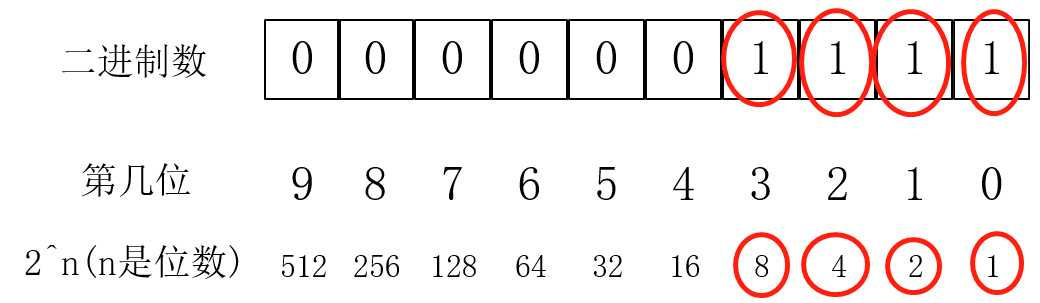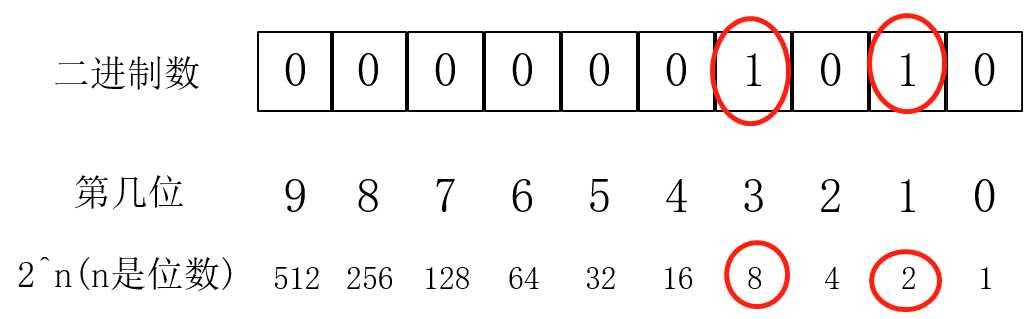1 //快速幂模板
2 int base = 5;  //base:底数
3 int m = 10;    //m:幂
4 int res = 1    //res:结果
5 while(m){
6     if(m % 2 == 1) res = res * base;
7     base = base * base;
8     m = m / 2;
9 }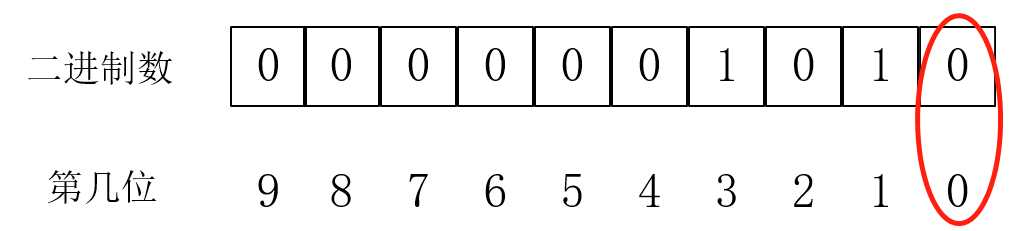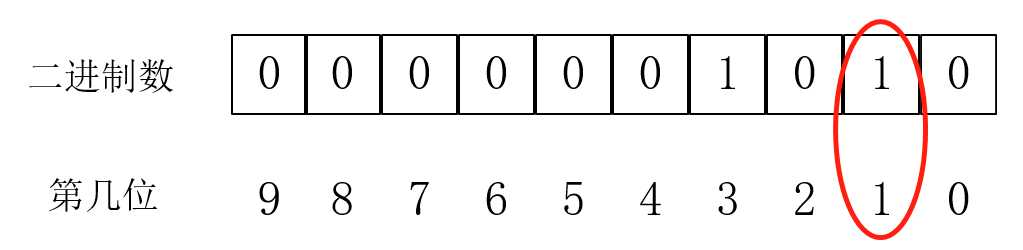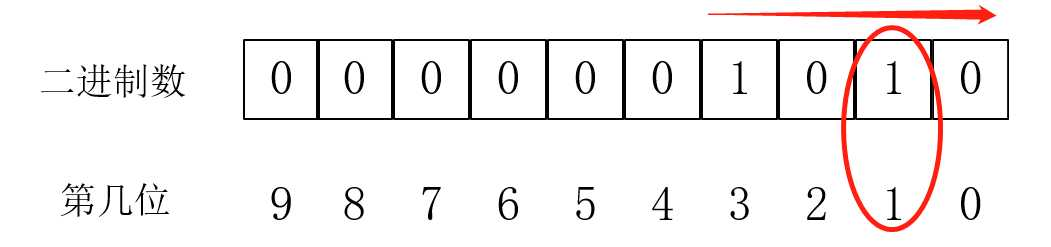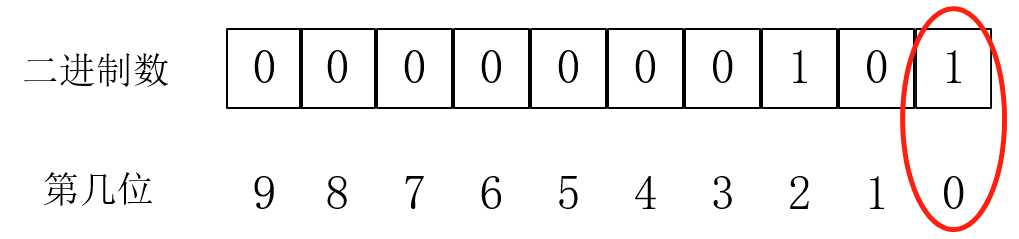1 //快速幂模板
2 int base = 5;  //base:底数
3 int m = 10;    //m:幂
4 int res = 1    //res:结果
5 while(m){
6     if(m & 1) res *= base;
7     base *= base;
8     m >>= 1;
9 }

(0)
(0)

© 2014 bubuko.com 版权所有 鲁ICP备09046678号-4